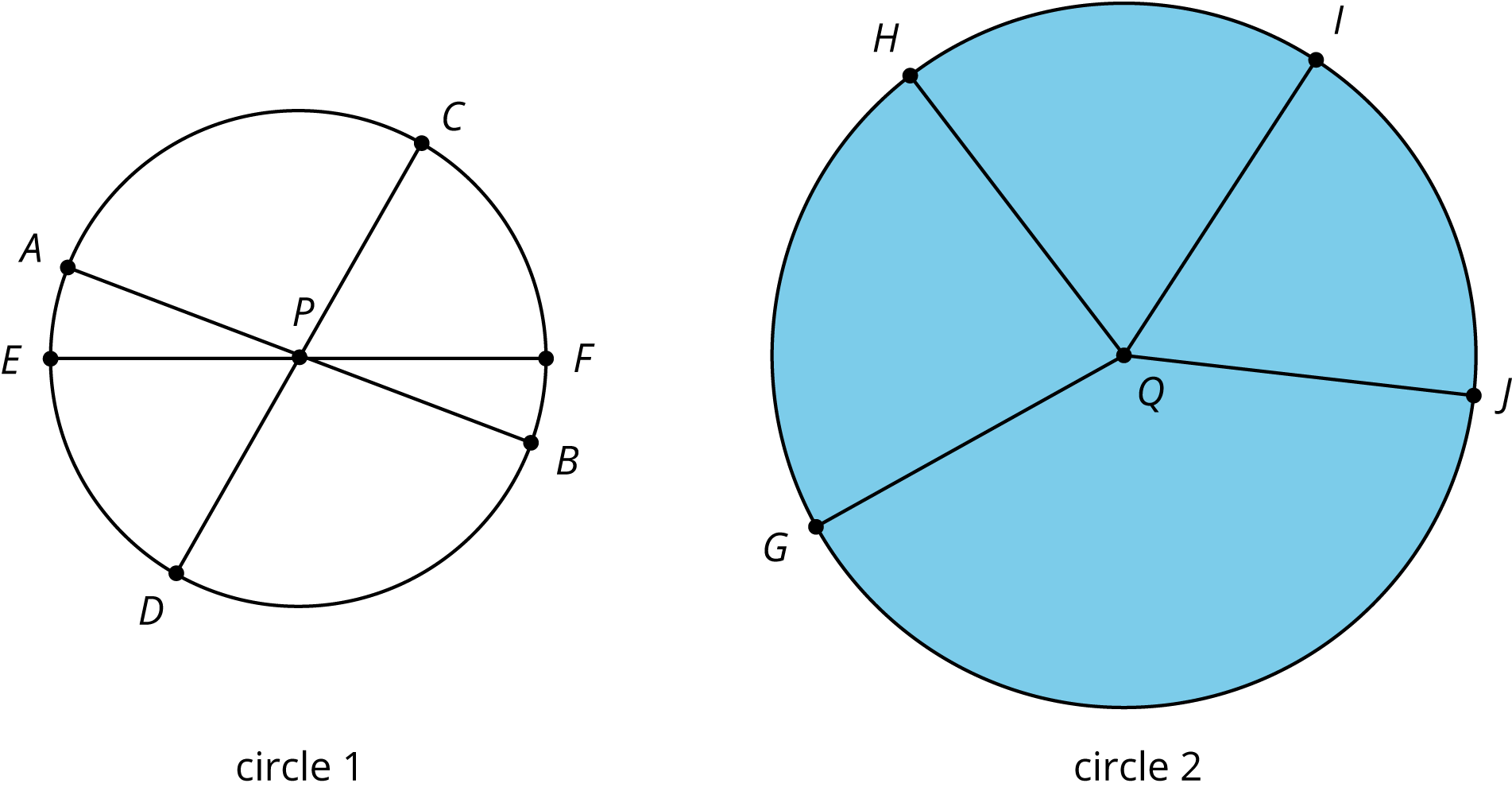# Mathematics Support

Math 7 | Unit 1 2 3 4 5 6 7 8 9

### Measuring Circles

Circumference of a Circle

Area of a Circle

## Circumference of a Circle

This week your student will learn why circles are different from other shapes, such as triangles and squares. Circles are perfectly round because they are made up of all the points that are the same distance away from a center.• This line segment from the center to a point on the circle is called the radius. For example, the segment from P to F is a radius of circle 1.
• The line segment between two points on the circle and through the center is called the diameter. It is twice the length of the radius. For example, the segment from E to F is a diameter of circle 1. Notice how segment EF is twice as long as segment PF.
• The distance around a circle is called the circumference. It is a little more than 3 times the length of the diameter. The exact relationship is C=πd, where π is a constant with infinitely many digits after the decimal point. One common approximation for π is 3.14.

We can use the proportional relationships between radius, diameter, and circumference to solve problems.

A cereal bowl has a diameter of 16 centimeters.

1. What is the radius of the cereal bowl?
1. 5 centimeters
2. 8 centimeters
3. 32 centimeters
4. 50 centimeters

2. What is the circumference of the cereal bowl?
1. 5 centimeters
2. 8 centimeters
3. 32 centimeters
4. 50 centimeters

Solution:

1. B, 8 centimeters. The diameter of a circle is twice the length of the radius, so the radius is half the length of the diameter. We can divide the diameter by 2 to find the radius. 16÷2=8.
2. D, 50 centimeters. The circumference of a circle is π times the diameter. 163.1450.

## Area of a Circle

This week your student will solve problems about the area inside circles. We can cut a circle into wedges and rearrange the pieces without changing the area of the shape. The smaller we cut the wedges, the more the rearranged shape looks like a parallelogram.The area of a circle can be found by multiplying half of the circumference times the radius. Using C=2πr we can represent this relationship with the equation:

A=12(2πr)r

Or

A=πr2

This means that if we know the radius, we can find the area. For example, if a circle has radius 10 cm, then the area is about 314 cm2, because 3.14102=314. We can also say that the area is 100π cm2.

A rectangular wooden board, 20 inches wide and 40 inches long, has a circular hole cut out of it.

1. The diameter of the circle is 6 inches. What is the area?
2. What is the area of the board after the circle is removed?

Solution:

1. 9π or about 28.26 in2. The radius of the hole is half of the diameter, so we can divide 6÷2=3. The area of a circle can be calculated A=πr2. For a radius of 3, we get 32=9. We can write 9π or use 3.14 as an approximation of pi, 3.149=28.26.
2. 8009π or about 771.74 in2. Before the hole was cut out, the entire board had an area of 2040 or 800 in2. We can subtract the area of the missing part to get the area of the remaining board, 80028.26=771.74.

IM 6–8 Math was originally developed by Open Up Resources and authored by Illustrative Mathematics, and is copyright 2017-2019 by Open Up Resources. It is licensed under the Creative Commons Attribution 4.0 International License (CC BY 4.0). OUR's 6–8 Math Curriculum is available at https://openupresources.org/math-curriculum/.

Disclaimer: This site provides external links and videos as a convenience and for informational purposes only. The appearance of external hyperlinks on the MCPS Family Mathematics Support Center website does not constitute an endorsement by the Montgomery County Public School System of any of the products or opinions contained therein.